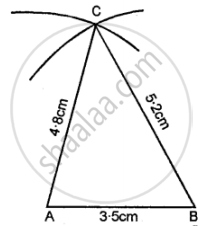# Construct Traingle Abc, When : Ab = 3.5 Cm, Ac = 4.8 Cm and Bc = 5.2 Cm. - Mathematics

Diagram
Sum

Construct traingle ABC, when : AB = 3.5 cm, AC = 4.8 cm and BC = 5.2 cm.

#### Solution

Steps of Construction:

(1) Draw a line AB = 3.5 cm.

(2) Using compasses and taking B as centre, draw an arc of 5.2 cm radius.

(3) With A as centre, draw an arc of 4.8.(4) Join AC and BC

Concept: Classification of Triangles (On the Basis of Sides, and of Angles)
Is there an error in this question or solution?

#### APPEARS IN

Selina Class 6 Mathematics
Chapter 26 Triangles (Including Types, Properties and Constructions)
Exercise 26 (B) | Q 2